Courses

# Flow Through Branched Pipes Notes | EduRev

## Civil Engineering (CE) : Flow Through Branched Pipes Notes | EduRev

The document Flow Through Branched Pipes Notes | EduRev is a part of the Civil Engineering (CE) Course Fluid Mechanics.
All you need of Civil Engineering (CE) at this link: Civil Engineering (CE)

## Flow Through Branched Pipes

In several practical situations, flow takes place under a given head through different pipes jointed together either in series or in parallel or in a combination of both of them.

## Pipes in Series

• If a pipeline is joined to one or more pipelines in continuation, these are said to constitute pipes in series. A typical example of pipes in series is shown in Fig. 36.1. Here three pipes A, B and are joined in series.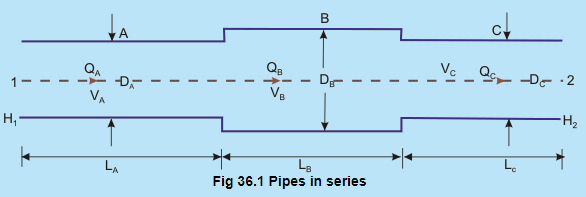n this case, rate of flow remains same in each pipe. Hence,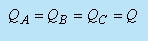If the total head available at Sec. 1 (at the inlet to pipe A) is  Hwhich is greater than H2 ,    the total head at Sec. 2 (at the exit of pipe C), then the flow takes place from 1 to 2 through the system of pipelines in series.

• Application of Bernoulli's equation between Secs.1 and 2 gives

H1 - H =   hf

where,  his the loss of head due to the flow from 1 to 2. Recognizing the minor and major losses associated with the flow, h can be written as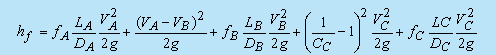(36.1)

Friction loss Loss due to
enlargement at
entry to pipe B
in pipe AFriction loss
in pipe B

The subscripts A, B and refer to the quantities in pipe A, B and C respectively. Cc is the coefficient of contraction.

• The flow rate Q satisfies the equation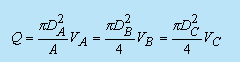Velocities VA, VB and VC in Eq. (36.1) are substituted from Eq. (36.2), and we get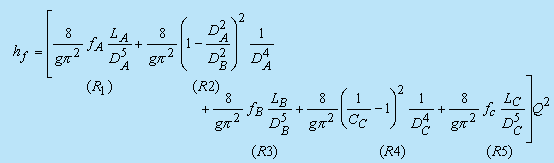(36.3)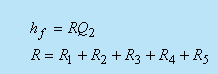(36.4)

Equation (36.4) states that the total flow resistance is equal to the sum of the different resistance components. Therefore, the above problem can be described by an equivalent electrical network system as shown in Fig. 36.2.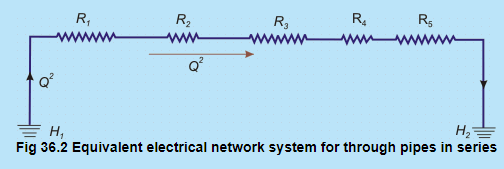Pipes In Parallel

• When two or more pipes are connected, as shown in Fig. 36.3, so that the flow divides and subsequently comes together again, the pipes are said to be in parallel.
• In this case (Fig. 36.3), equation of continuity gives

Q =  QA + QB                            (36.5)

where, is the total flow rate and QA  And Q are the flow rates through pipes and Brespectively.

• Loss of head between the locations 1 and 2 can be expressed by applying Bernoulli's equation either through the path 1-A-2 or 1-B-2.
• Therefore, we can write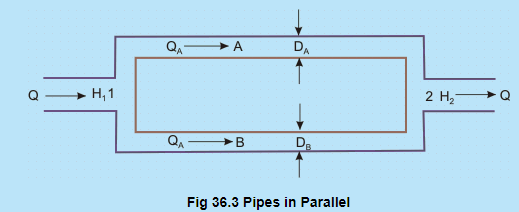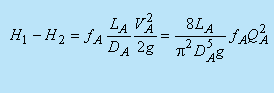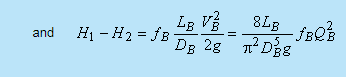Equating the above two expressions, we get -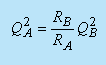(36.6)

where,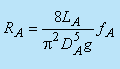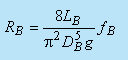Equations (36.5) and (36.6) give -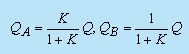(36.7)

Where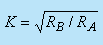(36.8)

The flow system can be described by an equivalent electrical circuit as shown in Fig. 36.4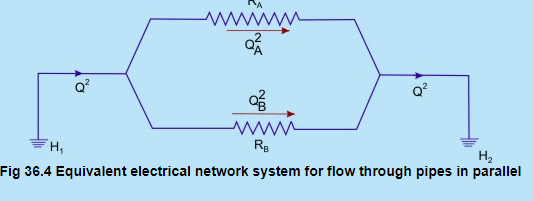From the above discussion on flow through branched pipes (pipes in series or in parallel, or in combination of both), the following principles can be summarized:

1. The friction equation must be satisfied for each pipe.
2. There can be only one value of head at any point.
3. Algebraic sum of the flow rates at any junction must be zero. i.e., the total mass flow rate towards the junction must be equal to the total mass flow rate away from it.
4. Algebraic sum of the products of the flux (Q2) and the flow resistance (the sense being determined by the direction of flow) must be zero in any closed hydraulic circuit.

The principles 3 and 4 can be written analytically as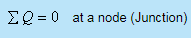(36.9)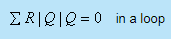(36.10)

While Eq. (36.9) implies the principle of continuity in a hydraulic circuit, Eq. (36.10) is referred to as pressure equation of the circuit.

Pipe Network: Solution by Hardy Cross Method

• The distribution of water supply in practice is often made through a pipe network comprising a combination of pipes in series and parallel. The flow distribution in a pipe network is determined from Eqs(36.9) and (36.10).
• The solution of Eqs (36.9) and (36.10) for the purpose is based on an iterative technique with an initial guess in Q
• The method was proposed by Hardy-Cross and is described below:
• The flow rates in each pipe are assumed so that the continuity (Eq. 36.9) at each node is satisfied. Usually the flow rate is assumed more for smaller values of resistance and vice versa.
• If the assumed values of flow rates are not correct, the pressure equation Eq. (36.10) will not be satisfied. The flow rate is then altered based on the error in satisfying the Eq. (36.10).
• Let Q0 be the correct flow in a path whereas the assumed flow be Q. The error din flow is then defined as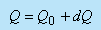(36.11)

Let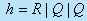(36.12a)

And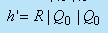(36.12b)

Then according to Eq. (36.10)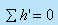in a loop                                         (36.13a)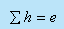in a loop                                              (36.13b)

Where 'e' is defined to be the error in pressure equation for a loop with the assumed values of flow rate in each path.
From Eqs (36.13a) and (36.13b) we have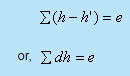(36.14)

Where dh (= h - h' ) is the error in pressure equation for a path. Again from Eq. (36.12a), we can write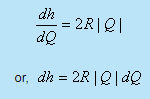(36.15)

Substituting the value of dh from Eq. (36.15) in Eq. (36.14) we have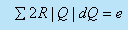Considering the error dQ to be the same for all hydraulic paths in a loop, we can write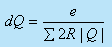(36.16)

he Eq. (36.16) can be written with the help of Eqs (36.12a) and (36.12b) as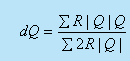(36.17)

The error in flow rate dis determined from Eq. (36.17) and the flow rate in each path of a loop is then altered according to Eq. (36.11).

The Hardy-Cross method can also be applied to a hydraulic circuit containing a pump or a turbine. The pressure equation (Eq. (36.10)) is only modified in consideration of a head source (pump) or a head sink (turbine) as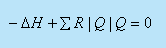(36.18)

where ΔH  is the head delivered by a source in the circuit. Therefore, the value of  ΔH  to be substituted in Eq. (36.18) will be positive for a pump and negative for a turbine.

Offer running on EduRev: Apply code STAYHOME200 to get INR 200 off on our premium plan EduRev Infinity!

## Fluid Mechanics

43 videos|70 docs|72 tests

,

,

,

,

,

,

,

,

,

,

,

,

,

,

,

,

,

,

,

,

,

;